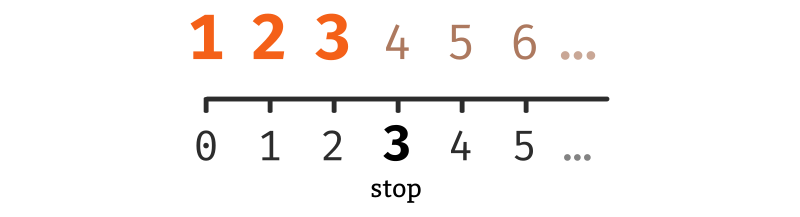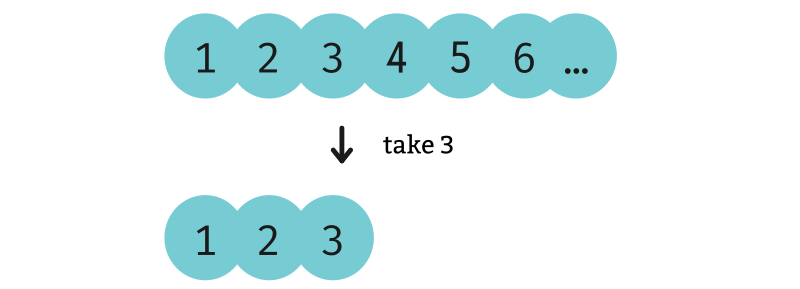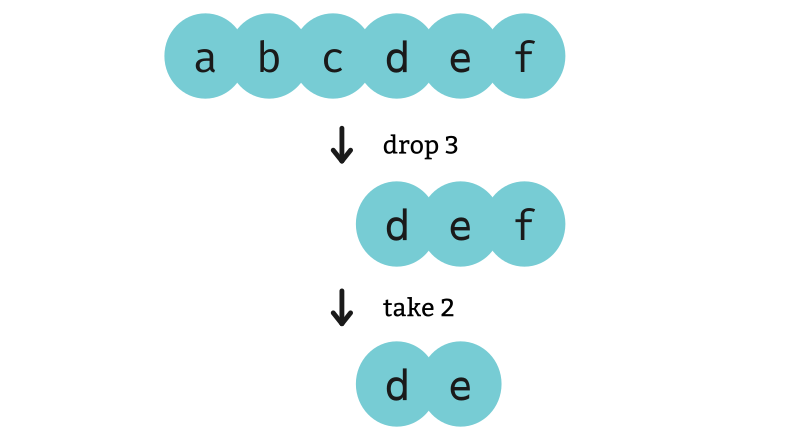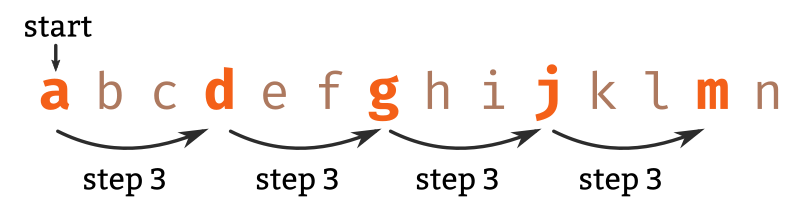# Iterator slicing

We discussed in the iterators lesson how Python iterators relate to Haskell lists. If you’re familiar with the `itertools` module, these next few lessons are to help you get started quickly with Haskell’s `Data.List` module. We will also discuss a few of Python’s built-in functions. All of the functions that we discuss here return iterators.

In all of the Python code examples, we assume that everything from `itertools` is imported:

``from itertools import *``

We’ll start by introducing `islice`, because we’ll need to use `islice` to demonstrate some of the other `itertools` functions.

## The `stop` parameter

The primary use of `islice` is to hack an iterator down to some fixed length.

``````>>> it = islice('abcdef', 3)

>>> ''.join(it)
'abc'``````

This is particularly important because some iterators never stop.

``````def to_infinity():
i = 1
while True:
yield i
i = i + 1``````

So when we want to print the contents of an iterator we’ll often need to print just some small chunk of its beginning.

``````>>> it = to_infinity()

>>> list(islice(it, 3))
[1, 2, 3]``````The equivalent Haskell function is `take`.

``````λ> toInfinity = enumFrom 1

λ> take 3 toInfinity
[1,2,3]```````take` is defined in the `Data.List` module in the `base` package, and it is also in `Prelude` (so it doesn’t need to be imported). This is the case for most of the Haskell functions that we discuss here.

## The `start` parameter

When `islice` is used with two arguments, the first argument is the (zero-indexed) position to start at, and the second argument is the position to stop before.

``````>>> it = islice('abcdef', 3, 5)

>>> ''.join(it)
'de'``````

In this example, the first argument `3` signifies that we want to start at the fourth character (`d`), and the second argument `5` specifies that we want to stop before the sixth character, so the last character we take is the fifth one (`e`).Haskell does not overload functions by arity like Python does. Instead we have a separate function, `drop`, `drop` in `Data.List` and `Prelude` which skips some number of elements and returns the remainder of the list. By using `drop` and `take` together, we can achieve the same effect as the two-argument version of `islice`.

``````λ> take 2 (drop 3 "abcdef")
"de"``````We might also consider writing this using the function composition operator `(.)`:

``````λ> (take 2 . drop 3) "abcdef"
"de"``````

## The `step` parameter

The final parameter lets us take elements periodically. In this example, we take the first element (`start = 0`) and every third element after that (`step = 3`).

``````>>> it = islice('abcdefghijklmn', 0, None, 3)

>>> ''.join(it)There is no direct analogue in the Haskell base package for this, so let’s think about how we might write one. We wouldn’t write this sort of function recursively in Python because it is too easy to overflow the call stack. Haskell does not suffer this same problem.

A lot of functions over lists can be expressed naturally with recursion, and this one is no exception.

``````startStep _     _    []       = []                                  -- 1
startStep 0     step (x : xs) = x : startStep (step - 1)  step xs   -- 2
startStep start step (x : xs) =     startStep (start - 1) step xs   -- 3``````
1. If the input list is empty, the output is empty.
2. If the `start` parameter is `0`, then the first element of the input list (`x`) is the first element of the output list.
3. Otherwise, we skip the first element of the input list, and apply `startStep` recursively with the `start` parameter reduced by one.
``````λ> startStep 0 3 "abcdefghijklmn"